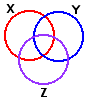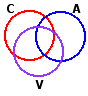Subchapter 3a.
Partial Correlation

Suppose you were to measure each of N subjects on each of three variables, X, Y, and Z, and find the following correlations:
 X versus Y: rXY = +.50 r2XY = .25 X versus Z: rXZ = +.50 r2XZ = .25 Y versus Z: rYZ = +.50 r2YZ = .25
For the moment, focus on the value of r2, which in each case (for this streamlined hypothetical example) is equal to .25. What this means is that for each pair of variables—XY, XZ, and YZ—the covariance, or variance overlap, is 25%. As illustrated in the following diagram, 25% of the variability of X overlaps with variability in Y; 25% of the variability of X overlaps with variability in Z; and 25% of the variability of Y also overlaps with variability in Z.Note as well that there is one region where all three of the variability circles overlap. The meaning of this three-way overlap is that a certain amount of the correlation found between any two of the variables is tied in with the correlation that each of those two has with the third. Thus, of the 25% variance overlap found between X and Y, approximately half (judging by the naked eye) is tied in with the overlaps that exist between XZ and YZ. Similarly for the 25% overlap between X and Z, where about half is bound up with the overlaps for XY and YZ. And similarly as well for the 25% overlap of YZ, where about half is tied up with the overlaps for XY and XZ.

Partial correlation is a procedure that allows us to measure the region of three-way overlap precisely, and then to remove it from the picture in order to determine what the correlation between any two of the variables would be (hypothetically) if they were not each correlated with the third variable. Alternatively, you can say that partial correlation allows us to determine what the correlation between any two of the variables would be (hypothetically) if the third variable were held constant. The partial correlation of X and Y, with the effects of Z removed (or held constant), would be given by the formula

 rXY·Z = rXY—(rXZ)(rYZ)sqrt[1—r2XZ] x sqrt[1—r2YZ]

which for the present example would work out as

 rXY·Z = .50—(.50)(.50)sqrt[1—.25] x sqrt[1—.25] rXY·Z = +.33 Hence r2XY·Z = .11

The same general structure would apply for calculating the partial correlation of X and Z, with the effects of Y removed:

 rXZ·Y = rXZ—(rXY)(rYZ)sqrt[1—r2XY] x sqrt[1—r2YZ]

and for for calculating the partial correlation of Y and Z, with the effects of X removed:

 rYZ·X = rYZ—(rXY)(rXZ)sqrt[1—r2XY] x sqrt[1—r2XZ]

Here is the apparatus of partial correlation applied to a real-life example. The Wechsler Adult Intelligence Scale (WAIS) is a device often used to measure "intelligence" beyond the years of childhood. Among its several sub-scales are three labeled as C, A, and V. The "C" stands for "comprehension," which chiefly reflects the test-taker's ability to comprehend the meanings and implications of written passages. The "A" refers to the test-taker's ability to perform tasks that require arithmétic ability. And the "V" stands for "vocabulary," which as you might imagine is a measure that increases or decreases in accordance with the breadth of the test-taker's vocabulary within the domain of the language in which the test is constructed. The following table shows the correlations typically found among these three sub-scales.
 C versus A: rCA = +.49 r2CA = .24 C versus V: rCV = +.73 r2CV = .53 A versus V: rAV = +.59 r2AV = .35Here the overlaps are less evenly proportioned, although the logic is quite the same. Of the 24% variance overlap that occurs in the relationship between comprehension and arithmétic ability, a substantial portion reflects the fact that both of these variables are correlated with vocabulary. If we were to remove the effects of vocabulary from the relationship between C and A, the resulting partial correlation would be

 rCA·V = rCA—(rCV)(rAV)sqrt[1—r2CV] x sqrt[1—r2AV]
 rCA·V = .49—(.73)(.59)sqrt[1—.53] x sqrt[1—.35] rCA·V = +.11 Hence r2CA·V = .01

In brief: with the effects of vocabulary removed, the correlation between comprehension and arithmétic ability collapses down to hardly anything at all. The practical inference is that if we were to administer the WAIS to a sample of subjects who were homogeneous with respect to breadth of vocabulary, the correlation between their scores on the comprehension and arithmétic sub-scales would prove fairly scant, on the order of r=+.11 and r2=.01.

In most cases a partial correlation of the general form rXY·Z will turn out smaller than the original correlation rXY. In those cases where it turns out larger, the third variable, Z, is typically spoken of as a supressor variable on the assumption that it is suppressing the larger correlation that would appear between X and Y if Z were held constant.

Suppose, for example, that a rather cranky professor has just administered an exam in his statistics course, and that for each student in the course we have measures on each of the following three variables:

 X = the amount of effort spent on studying for the exam beforehand Y = the student's score on the exam Z = a measure of the degree to which the professor inspires fear and trembling in the student

And here are the correlations among the three variables:
 X versus Y: rXY = +.20 r2XY = .04 X versus Z: rXZ = +.80 r2XZ = .64 Y versus Z: rYZ = —.40 r2YZ = .16
Now isn't it odd that the correlation between X and Y should end up as a scant rXY=+.20 and r2XY=.04, indicating a mere 4% covariance between the degrees of effort that students put into to the exam and the scores that they receive on it? Examine the other two correlations, however, and you will see that it is not so odd after all. The greater the fear and trembling, the greater the effort that students tend to put into preparing for the exam; hence rXZ=+.80 and r2XZ=.64. On the other hand, the greater the fear and trembling, the less well students tend to do on the exam, as witness rYZ=.40 and r2YZ=.16. Remove the supressing effects of fear and trembling from the equation,
rXY·Z =
.20(.80)(.40)
sqrt[1.64] x sqrt[1.16]
 rXY=.20 r2XY=.04 rXY·Z=.95 r2XY·Z=.90
rXY·Z =
+.95
and the correlation between effort and exam score goes from a scant rXY=+.20 to an impressive rXY·Z=+.95. Or alternatively: remove the fear and trembling, and the covariance between effort and exam score goes from a mere 4% to a very substantial 90% (r2XY·Z=.90).

The VassarStats computational site includes a page that will calculate the partial correlation coefficients for any particular set of three intercorrelated variables.

End of Subchapter 3a.